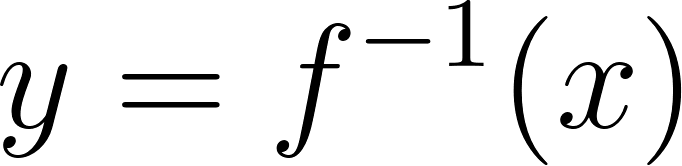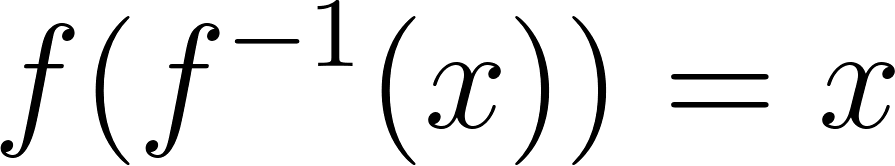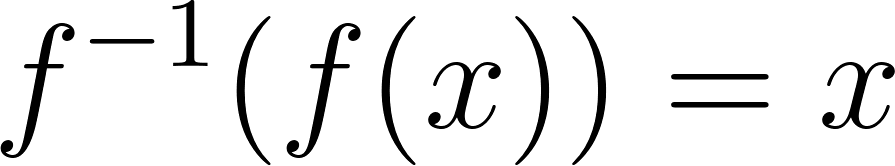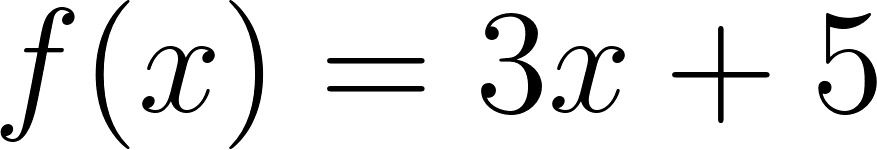×
Get Full Access to Calculus: Early Transcendentals - 1 Edition - Chapter 1.3 - Problem 19e
Get Full Access to Calculus: Early Transcendentals - 1 Edition - Chapter 1.3 - Problem 19e

×

# Answer: Finding inverse f unctions a. Find the inverse ofISBN: 9780321570567 2

## Solution for problem 19E Chapter 1.3

Calculus: Early Transcendentals | 1st Edition

• Textbook Solutions
• 2901 Step-by-step solutions solved by professors and subject experts
• Get 24/7 help from StudySoup virtual teaching assistantsCalculus: Early Transcendentals | 1st Edition

4 5 1 324 Reviews
29
4
Problem 19E

Finding  inverse function :

a) Finding  the inverse function (on the given interval, if specified)? and write  it in the.

b)Verify the relationshipsand..

Step-by-Step Solution:

Step 1 of 5

Given ,a)We need to findb)and.

Step 2 of 5

Step 3 of 5

##### ISBN: 9780321570567

The full step-by-step solution to problem: 19E from chapter: 1.3 was answered by , our top Calculus solution expert on 03/03/17, 03:45PM. Calculus: Early Transcendentals was written by and is associated to the ISBN: 9780321570567. The answer to “?Finding inverse function : a) Finding the inverse function (on the given interval, if specified)? and write it in the . b)Verify the relationships and . .” is broken down into a number of easy to follow steps, and 27 words. Since the solution to 19E from 1.3 chapter was answered, more than 372 students have viewed the full step-by-step answer. This full solution covers the following key subjects: Inverse, pecified, function, given, interval. This expansive textbook survival guide covers 85 chapters, and 5218 solutions. This textbook survival guide was created for the textbook: Calculus: Early Transcendentals, edition: 1.

Unlock Textbook Solution